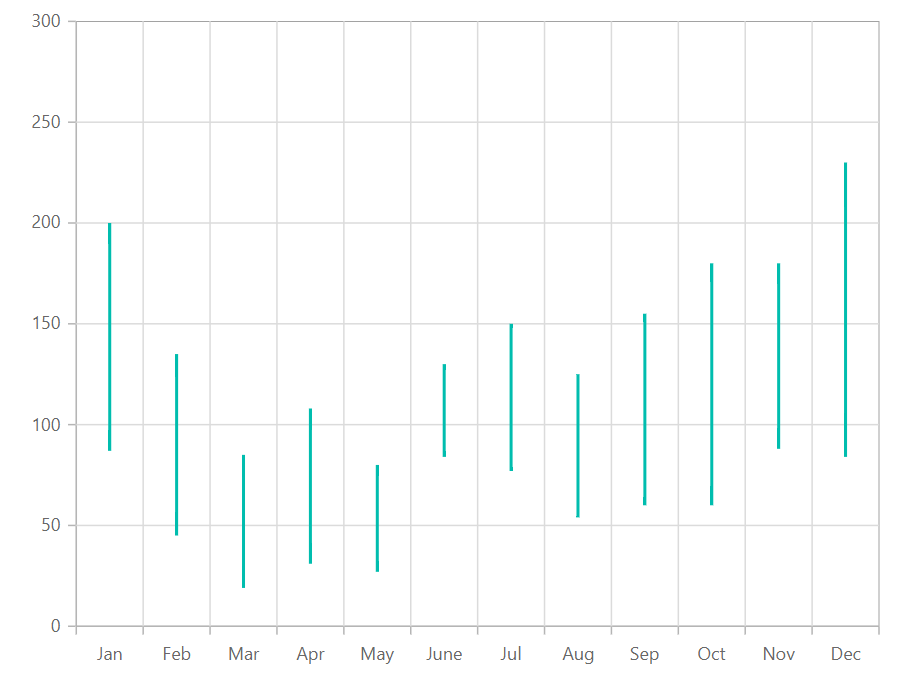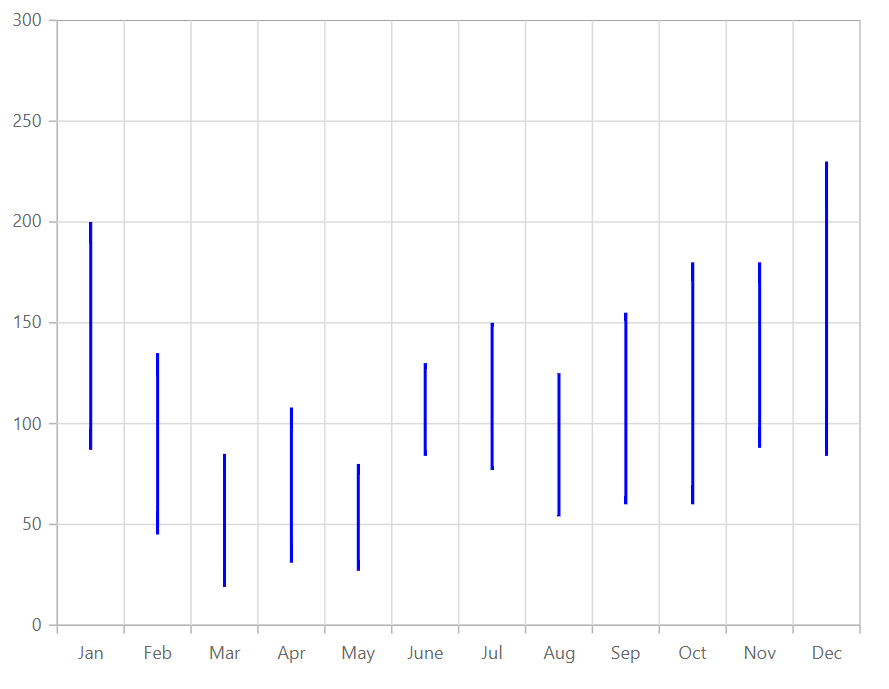# Hilo in Blazor Charts Component

11 Aug 20233 minutes to read

## Hilo

Hilo series illustrates the price movements in stock using the higher and lower values and it can be rendered by specifying the series Type as Hilo. Hilo series requires three fields (XName, High and Low) to show the higher and lower price in the stock.

``````@using Syncfusion.Blazor.Charts

<SfChart>
<ChartPrimaryXAxis ValueType="Syncfusion.Blazor.Charts.ValueType.Category"/>

<ChartSeriesCollection>
<ChartSeries DataSource="@StockDetails" XName="X" High="High" Low="Low" Type="ChartSeriesType.Hilo">
</ChartSeries>
</ChartSeriesCollection>
</SfChart>

@code{
public class Data
{
public string X { get; set; }
public double Y { get; set; }
public double High { get; set; }
public double Low { get; set; }
}

public List<Data> StockDetails = new List<Data>
{
new Data{ X= "Jan", Low= 87, High= 200 },
new Data{ X= "Feb", Low= 45, High= 135 },
new Data{ X= "Mar", Low= 19, High= 85 },
new Data{ X= "Apr", Low= 31, High= 108 },
new Data{ X= "May", Low= 27, High= 80 },
new Data{ X= "June",Low= 84, High= 130 },
new Data{ X= "Jul", Low= 77, High=150 },
new Data{ X= "Aug", Low= 54, High= 125 },
new Data{ X= "Sep", Low= 60, High= 155 },
new Data{ X= "Oct", Low= 60, High= 180 },
new Data{ X= "Nov", Low= 88, High= 180 },
new Data{ X= "Dec", Low= 84, High= 230 }
};
}``````NOTE

Refer to our Blazor Hilo Chart feature tour page to know about its other groundbreaking feature representations and also explore our Blazor Hilo Chart Example to know how to render and configure the Hilo type series.

## Series customization

The following properties can be used to customize the Hilo series.

• Fill – Specifies the color of the series.
• Opacity – Specifies the opacity of Fill.
``````@using Syncfusion.Blazor.Charts

<SfChart>
<ChartPrimaryXAxis ValueType="Syncfusion.Blazor.Charts.ValueType.Category" />

<ChartSeriesCollection>
<ChartSeries DataSource="@StockDetails" XName="X" High="High" Low="Low" Fill="blue" Type="ChartSeriesType.Hilo">
</ChartSeries>
</ChartSeriesCollection>
</SfChart>

@code{
public class Data
{
public string X { get; set; }
public double Y { get; set; }
public double High { get; set; }
public double Low { get; set; }
}

public List<Data> StockDetails = new List<Data>
{
new Data{ X= "Jan", Low= 87, High= 200 },
new Data{ X= "Feb", Low= 45, High= 135 },
new Data{ X= "Mar", Low= 19, High= 85 },
new Data{ X= "Apr", Low= 31, High= 108 },
new Data{ X= "May", Low= 27, High= 80 },
new Data{ X= "June",Low= 84, High= 130 },
new Data{ X= "Jul", Low= 77, High=150 },
new Data{ X= "Aug", Low= 54, High= 125 },
new Data{ X= "Sep", Low= 60, High= 155 },
new Data{ X= "Oct", Low= 60, High= 180 },
new Data{ X= "Nov", Low= 88, High= 180 },
new Data{ X= "Dec", Low= 84, High= 230 }
};
}``````NOTE

Refer to our Blazor Charts feature tour page for its groundbreaking feature representations and also explore our Blazor Chart Example to know various chart types and how to represent time-dependent data, showing trends at equal intervals.